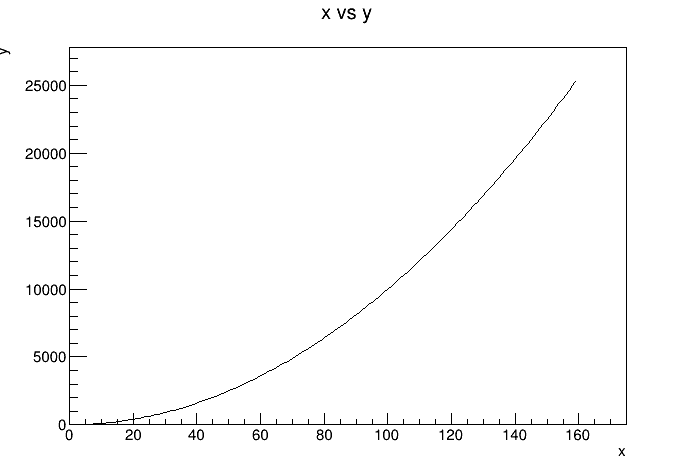# Df 0 2 1_Create T Graph¶

This tutorial shows how to fill a TGraph using the Dataframe.

Author: Enrico Guiraud, Danilo Piparo, Massimo Tumolo
This notebook tutorial was automatically generated with ROOTBOOK-izer from the macro found in the ROOT repository on Monday, July 06, 2020 at 11:37 AM.

In :
ROOT::EnableImplicitMT(2);

ROOT::RDataFrame d(160);


Create a trivial parabola

In :
auto dd = d.Alias("x", "rdfentry_").Define("y", "x*x");

auto graph = dd.Graph("x", "y");


This tutorial is ran with multithreading enabled. the order in which points are inserted is not known, so to have a meaningful representation points are sorted.

In :
graph->Sort();
auto c = new TCanvas();
graph->DrawClone("APL");


Draw all canvases

In :
gROOT->GetListOfCanvases()->Draw()